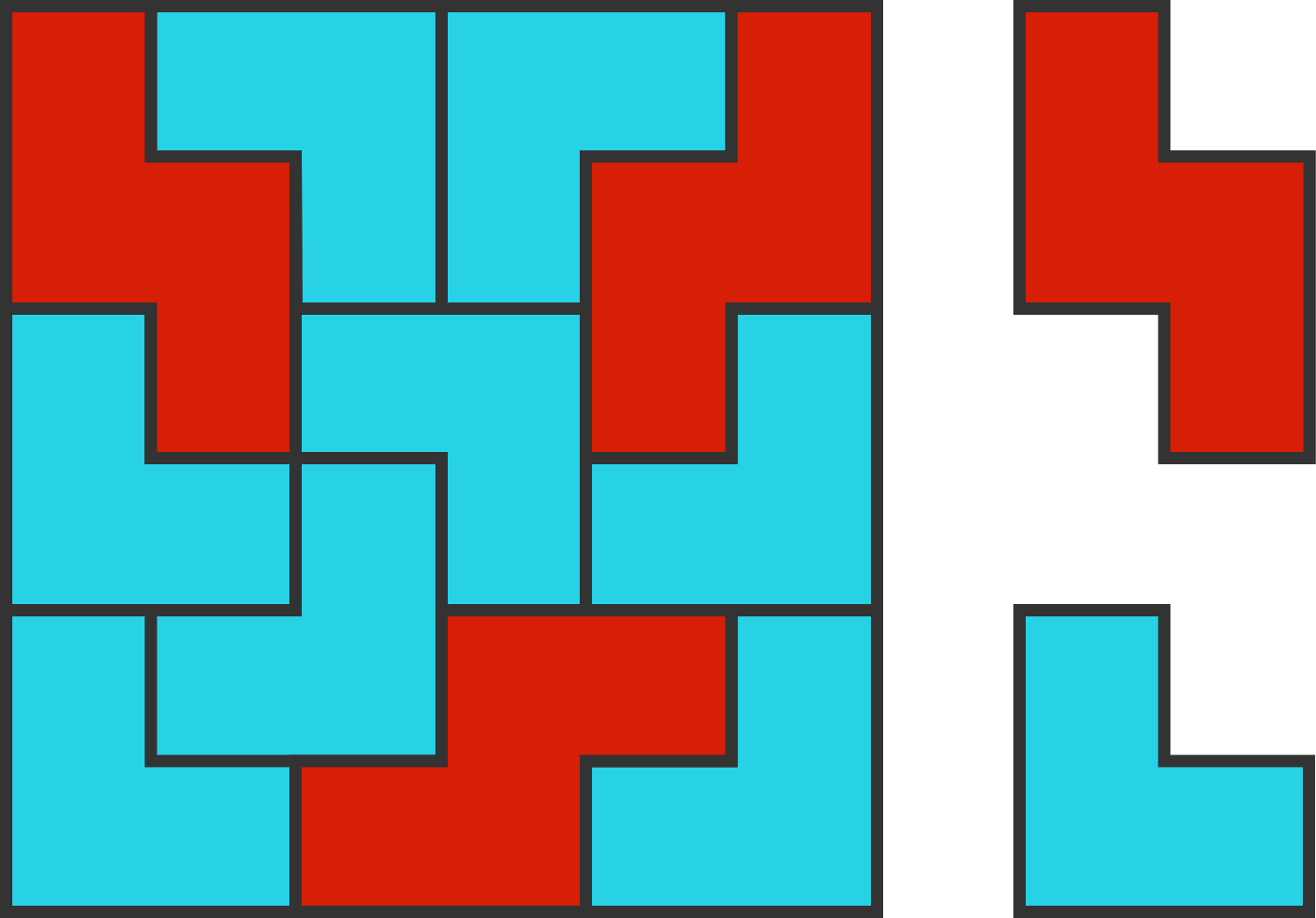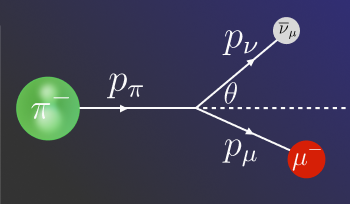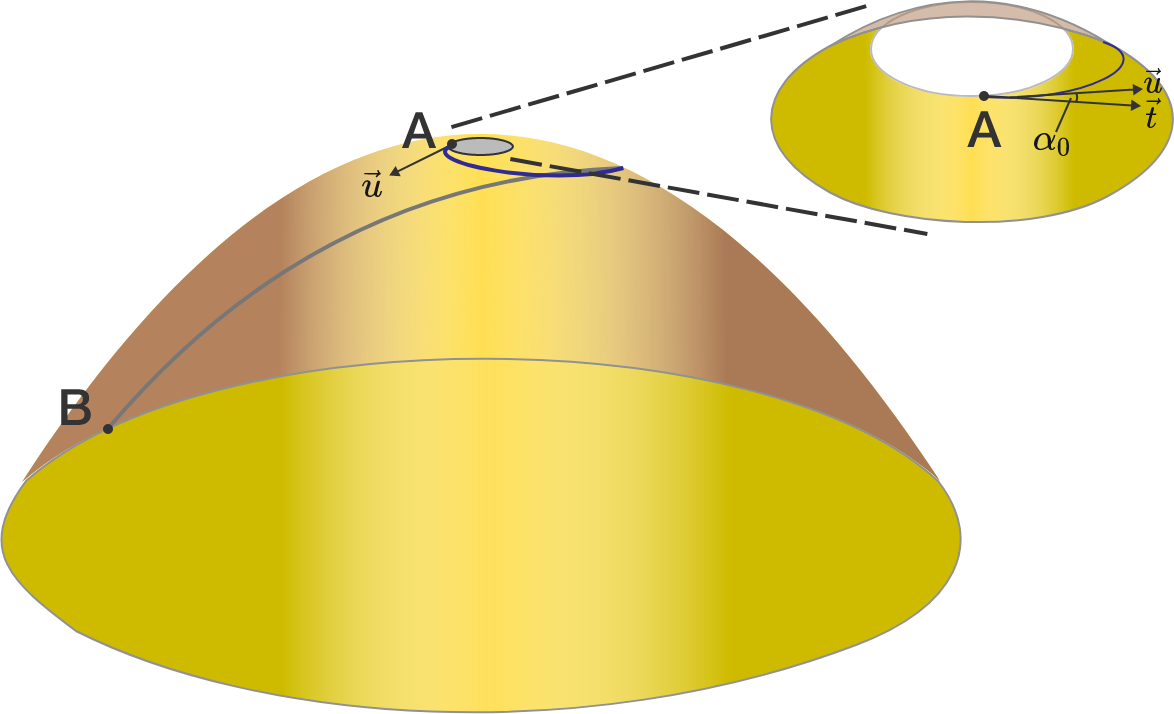# Problems of the Week

Contribute a problem

# 2018-07-16 AdvancedA figure is constructed from two parabolas with vertical axes of symmetry and distinct vertices. The parabolas intersect through each other's vertices. The figure is contained by horizontal segments that go through the parabolas' vertices.

What fraction of the figure's area is the area bounded by the two parabolas?

$\frac{17820}{495} = 36$

The above is called an “awesome fraction” because it equals a positive integer and uses each of the digits 0 through 9 exactly once on either side of the equal sign ($=$).

What is the sum of all integers that can be expressed as awesome fractions?

Note: All integers must be expressed without leading zeros. You may make use of the coding environment below:

You need to be connected to run codeThis $6 \times 6$ square has three Z-tiles (red) and eight V-tiles (blue).

You want to build a $9 \times 9$ square using these two types of tiles, both of which can be rotated and reflected.

What is the maximum number of Z-tiles you can use?

Neutrinos arrive at Earth's surface all the time. Energies below $\sim\SI{50}{\giga\electronvolt}$ are typical and result from the breakdown of other particles high in the atmosphere. But last fall, a neutrino arrived with an energy of $\SI{290}{\tera\electronvolt}$, about $20$ times more energetic than the most powerful collisions at the LHC. At the moment, our understanding of where these exceptional particles could come from is poor.

Evidence is pointing toward a blazar in the direction of constellation Orion, which comprises a galaxy that orbits a spinning, supermassive black hole (SMBH). Calculations suggest that such SMBH form powerful jets that eject matter along their axis of rotation. The idea is that a very high energy pion $\pi^{-}$ was accelerated in the jet before decaying into a neutrino $\overline{\nu}_\mu$ and a muon $\mu^-$, and that the neutrino sailed billions of years across the Universe, before landing at the IceCube detector in Antarctica.

Suppose a neutrino with energy $E_\nu$ is produced by a charged-pion decay process (which also produces a muon): $\pi^- \to \mu^- + \overline{\nu}_\mu.$Use conservation of momentum and conservation of energy to calculate the pion's energy $E_\pi$ at the maximum possible angle $\theta$ that sent the $\SI{290}{\tera\electronvolt}$ neutrino hurtling at Antarctica.

How many times larger is $E_\pi$ compared to $E_\nu?$

Note: At ultra-high energies, the relationship between a particle's energy $E$ and momentum $p$ is $E=\sqrt{p^2c^2+m^2c^4},$ where $m$ is the particle's mass $\big($instead of the classical relationship $E=p^2/2m\big).$

Assume the pion's mass $m_\pi = \SI{140}{\mega\electronvolt}/c^2$ and the muon's mass $m_\mu = \SI{106}{\mega\electronvolt}/c^2.$ The neutrino's mass is so small that you can assume it is $0.$

The surface of a volcano is represented by the frustum of the paraboloid $z = 225 - x^2 - y^2$ for $0 \le z \le 224.$ A geological research station is located at point $A=(1,0,224)$ on the edge of the crater of the volcano. The main research center for the volcano is located at point $B=(15,0,0).$

The scientists studying the volcano have established a path from $A$ to $B$ that passes all the way around the volcano exactly once—so that measurements can be taken of all sides of the volcano—and has the shortest possible length just in case the volcano shows signs of erupting.

What is the angle $\alpha_0$ that the tangent vector $\mathbf{t}$ to the path makes with the horizontal tangent vector $\mathbf{u}$ to the surface of the volcano at point $A?$ Give your answer in degrees, correct to $1$ decimal place.

Note: This integral will (in)definitely help.×

Problem Loading...

Note Loading...

Set Loading...